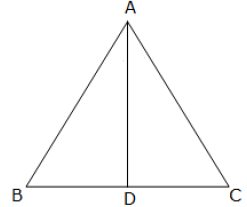"
">

# In $\triangle A B C, A D \perp B C$ and $A D^{2}=B D . C D$.Prove that $\angle B A C=90^o$."

Given:

In $\triangle ABC$, $AD$ is perpendicular to $BC$ and $AD^2 = BD.DC$.

To do:

We have to prove that $\angle BAC = 90^o$.

Solution:

In right angled triangles $ADB$ and $ADC$

Using Pythagoras theorem, we get,

$AB^2 = AD^2 + BD^2$..............(i)

$AC^2 = AD^2 + DC^2$............(ii)

From (i) and (ii), we get,

$AB^2 + AC^2 = 2AD^2 + BD^2 + DC^2$

$\Rightarrow AB^2 + AC^2 = 2BD.CD + BD^2 + CD^2$         [Since $AD^2 = BD.CD$]

$\Rightarrow AB^2 + AC^2 = (BD + CD)^2$

$\Rightarrow AB^2 + AC^2 = BC^2$             [Since $BC=BD+CD$]

This implies,

$\triangle ABC$ is a right angled triangle with right angle at $A$

$\Rightarrow \angle BAC = 90^o$

Hence proved.

Updated on: 10-Oct-2022

69 Views